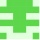Home Communities
IT Knowledge
Inspiration
Languages
EN

# C# / .NET - replace first n characters in string (range operator ..)

3 points
Created by:aaliyah
411

In this article, we would like to show you how to replace the first n characters in a string in C# / .NET.

Quick solution:

``````int n = 2;
string text = "ABCD";
string replacement = "xy";
string result = replacement + text[n..];

Console.WriteLine(result);  // xyCD``````

Note: range operator `..` was introduced in C# 8.0.

## 1. Practical examples

### 1.1 With `+` and range operator

In this example, we will take the last `n` characters from the original string and add them to the end of the replacement.

``````using System;

public class StringUtils
{
public static void Main(string[] args)
{
int n = 2;
string text = "ABCD";
string replacement = "xy";
string result = replacement + text[n..];

Console.WriteLine(result);  // xyCD
}
}``````

### 1.2 With `string.Concat()` method and range operator

This approach is equivalent to the above one. Instead of `+` operator we concatenate the strings using `string.Concat()` method.

``````using System;

public class StringUtils
{
public static void Main(string[] args)
{
int n = 2;
string text = "ABCD";
string replacement = "xy";
string result = string.Concat(replacement, text[n..]);

Console.WriteLine(result);  // xyCD
}
}``````

## 2. Practical example using string `Regex.Replace()` with regex pattern

In this example, we use `Regex.Replace()` with `"^.{n}"` regex to replace the first 2 characters in the `text` string.

Regex explanation:

• `^` - matches the beginning of the string,
• `.` - matches any character except linebreaks,
• `{n}` - matches the specified quantity of the previous token (in our case the `.`).
``````using System;
using System.Text.RegularExpressions;

public class StringUtils
{
public static void Main(string[] args)
{
int n = 2;
string text = "ABCD";
string replacement = "xy";
string pattern = @"^.{" + n + "}";

string result = Regex.Replace(text, pattern, replacement);

Console.WriteLine(result);  // xyCD
}
}``````
Join to our subscribers to be up to date with content, news and offers.